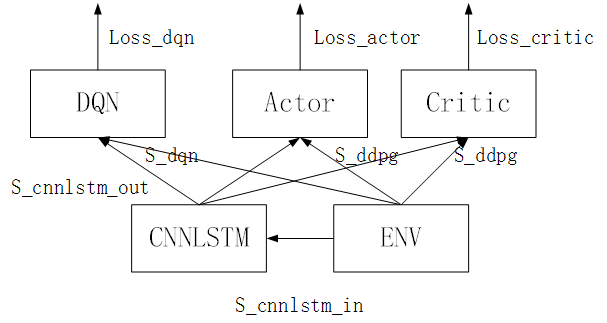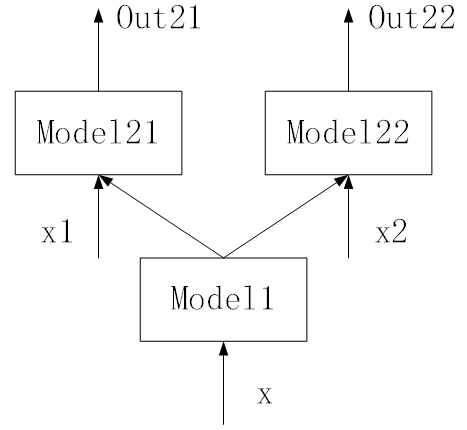# Can not create a new calculation graph after the backward propagation

#1

Background：I use DQN and DDPG to solve two tasks simultaneously. The `state(input)` of DQN and DDPG are both two parts. One part is the states of the environment, and the other one is the states abstracted from the environment by CNN+LSTM. The two parts are concatenate in `forward_dqn()` , `forward_actor()` and `forward_critic()` respectively.

Question1： I backward propagate the `loss_dqn` , `loss_ddpg_actor` , and `loss_ddpg_critic` in sequence and get the error “Trying to backward through the graph a second time, but the buffers have already been freed.” in the backward propagation of `loss_ddpg_actor` . Since after the backward propagation of loss_dqn, the computational graph has been freed, so I have forward propagated CNN+LSTM again to calculate the loss_ddpg_actor. Why the computational graph cannot be created again? Thanks.Model: (env: environment)

``````output_cnnlstm = cnnlstm.forward(env)
DQN_output = dqn.forward(cat(output_cnnlstm, state_env))
Actor_output = actor.forward(cat(output_cnnlstm, state_env))
Critic_output = critic.forward(cat(output_cnnlstm, state_env))
``````

Code 1 (Q1):

``````    # dqn
# forward: cnnlstm
s_cnnlstm_out, _, _ = self.model.forward_cnnlstm(s_cnnlstm, flag_optim=True)
# forward: dqn
q_eval_dqn = self.model.forward_dqn_eval(s_dqn, s_cnnlstm_out).gather(1, a_dqn)
q_next_dqn = self.model.forward_dqn_target(s_dqn_next, s_cnnlstm_out).detach()
q_target_dqn = r + GAMMA_DQN * q_next_dqn.max(dim=1).reshape(SIZE_BATCH * SIZE_TRANSACTION, 1)
# optimzie: dqn
loss_dqn = self.loss_dqn(q_eval_dqn, q_target_dqn)
self.optimizer_cnnlstm.zero_grad()
self.optimizer_dqn.zero_grad()
loss_dqn.backward()
self.optimizer_cnnlstm.step()
self.optimizer_dqn.step()
loss_dqn = loss_dqn.detach().numpy()
# ddpg
# actor
# forward: cnnlstm
s_cnnlstm_out, _, _ = self.model.forward_cnnlstm(s_cnnlstm, flag_optim=True)
# forward: ddpg: actor
a_eval_ddpg = self.model.forward_actor_eval(s_ddpg, s_cnnlstm_out)
# optimze: ddpg: cnnlstm + actor
loss_ddpg_actor = - self.model.forward_cirtic_eval(s_ddpg, a_eval_ddpg, s_cnnlstm_out).mean()
self.optimizer_cnnlstm.zero_grad()
self.optimizer_actor.zero_grad()
loss_ddpg_actor.backward()
self.optimizer_cnnlstm.step()
self.optimizer_actor.step()
loss_ddpg_actor = loss_ddpg_actor.detach().numpy()
``````

Question2: I write a demo to test the propagation process and the demo seems to work well since the loss descends normally and the test error is low. So I want to ask the difference between the two codes and models.

Model:``````output_model1 = model1.forward(x)
output_model21 = model21.forward(cat(output_model1, x1))
output_model22 = model221.forward(cat(output_model1, x2))
``````

compared with the model of Q1, output_model1 ~ cnnlstm, output_model21 ~ DQN, output_model22 ~ Actor

Question3： I set breakpoint in the demo after `loss1.backward()` and before `optimizer1.step()` . However, on the one hand, the weight of the linear layer of Model21 changes with the optimization. On the other hand, `x._grad` is a gradient value tensor, while `x1._grad` is `None` . So I wonder the parameters of Model21 are optimized whether or not and why `x1._grad` is None.

Code 2 (Q2 and Q3):

``````for i in range(NUM_OPTIM):
# optimize task 1
y1_pred = self.model.forward_task1(x, x1)
loss1 = self.loss_21(y1_pred, y1)
self.optimizer1.zero_grad()
self.optimizer21.zero_grad()
loss1.backward()
self.optimizer1.step()
self.optimizer21.step(
# optimze task 2
y2_pred = self.model.forward_task2(x, x2)
loss2 = self.loss_22(y2_pred, y2)
self.optimizer1.zero_grad()
self.optimizer22.zero_grad()
loss2.backward()
self.optimizer1.step()
self.optimizer22.step()
``````
#2

My fault. I forget to `detach()` the hidden state and the cell state of LSTM from the computational graph in `self.model.forward_cnnlstm`.